Ex 14.2

Chapter 14 Class 11 Probability
Serial order wise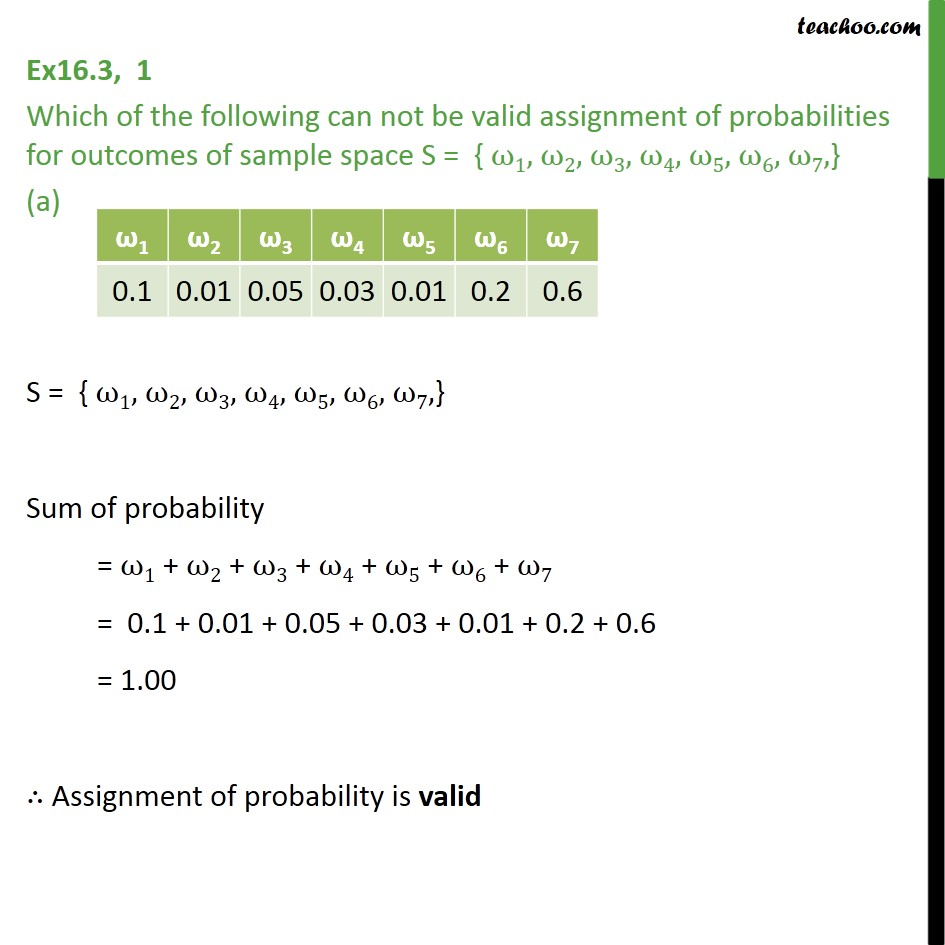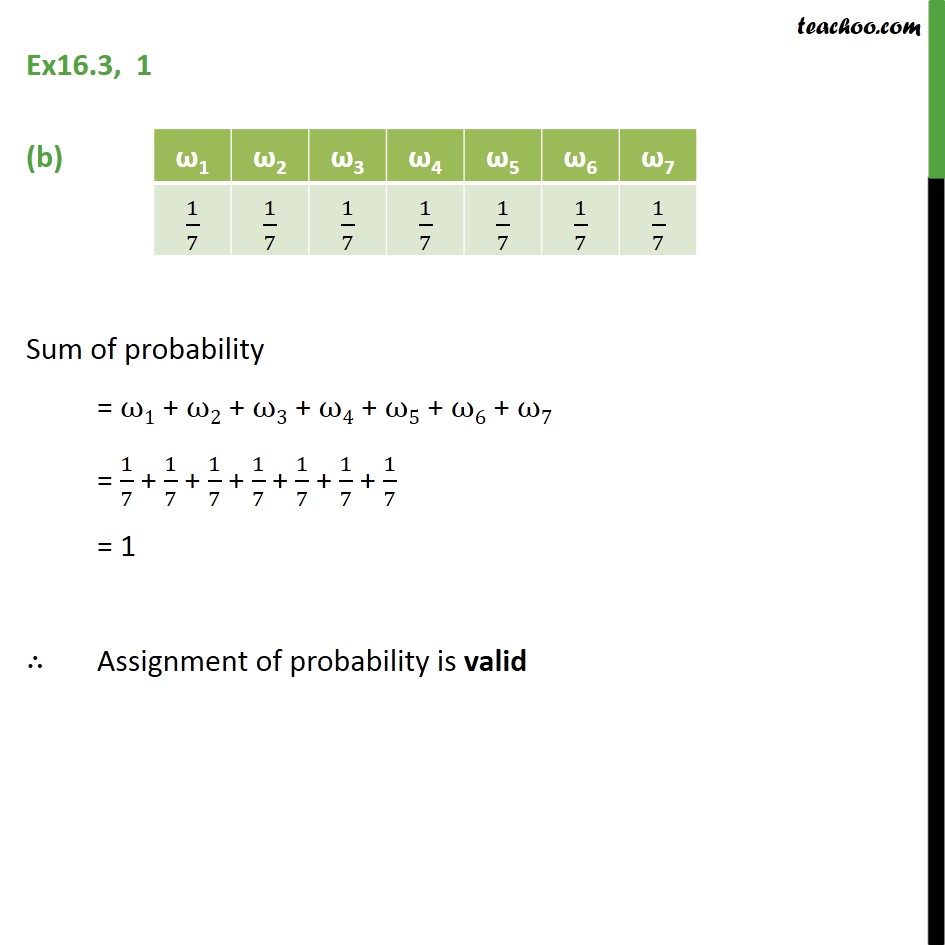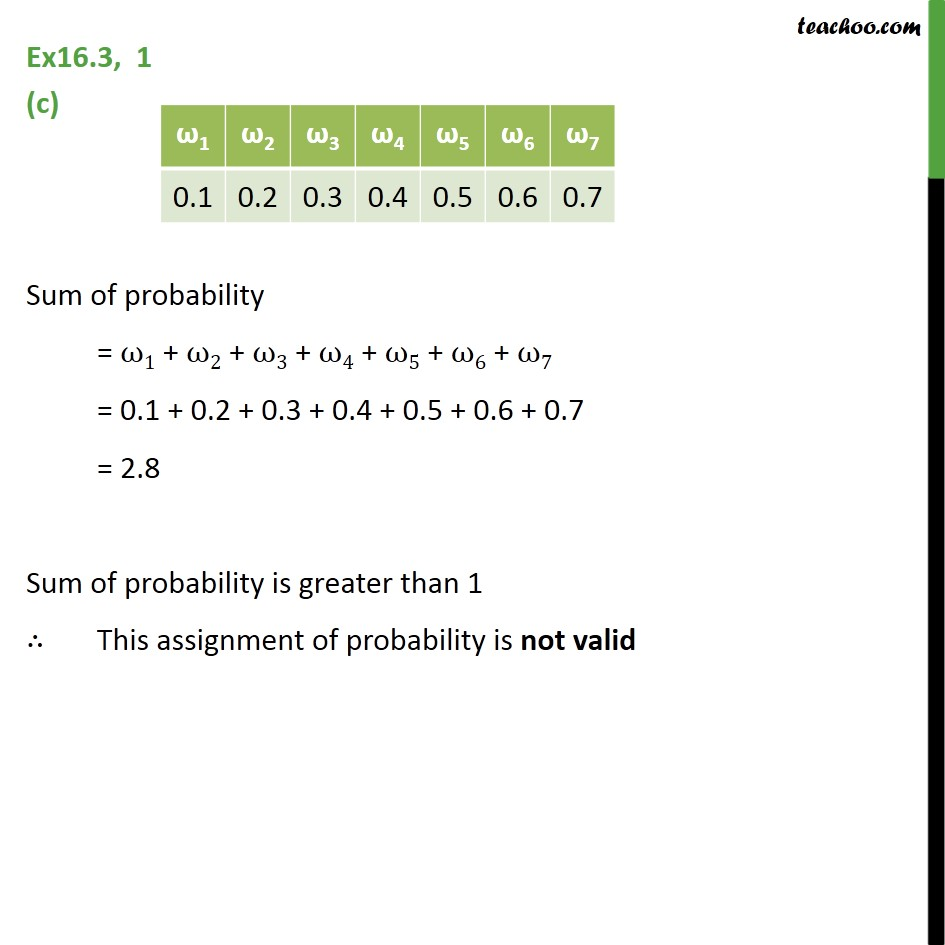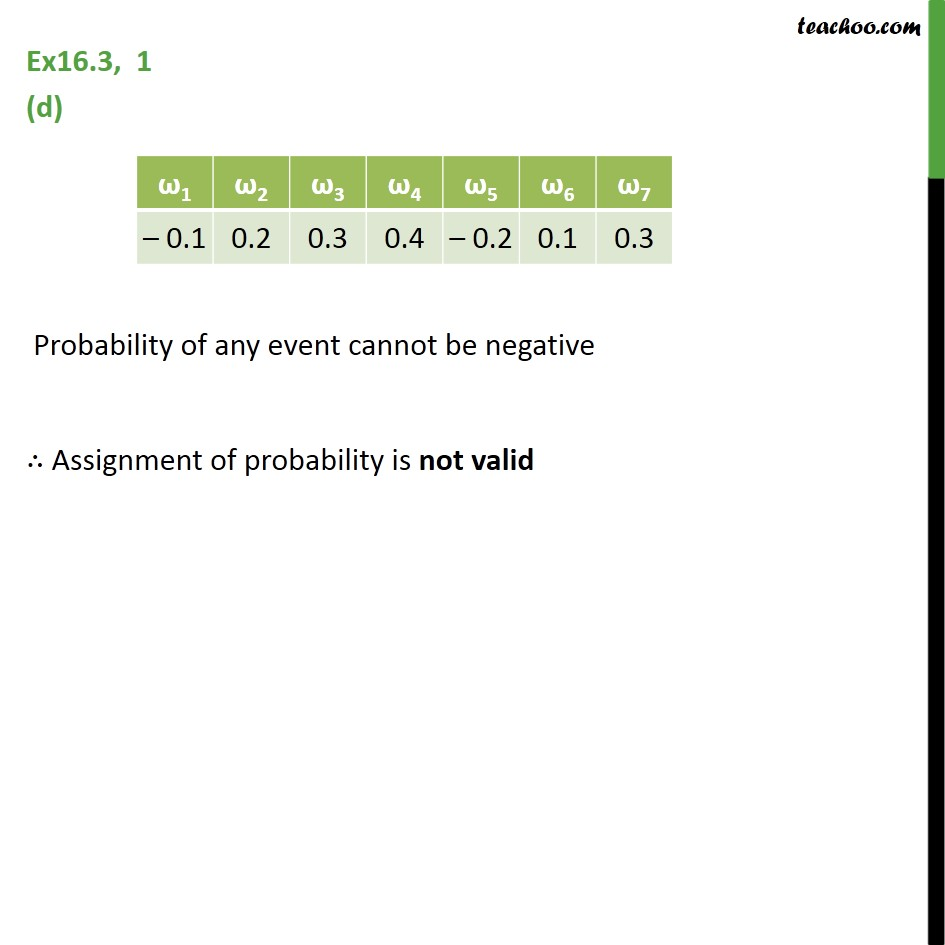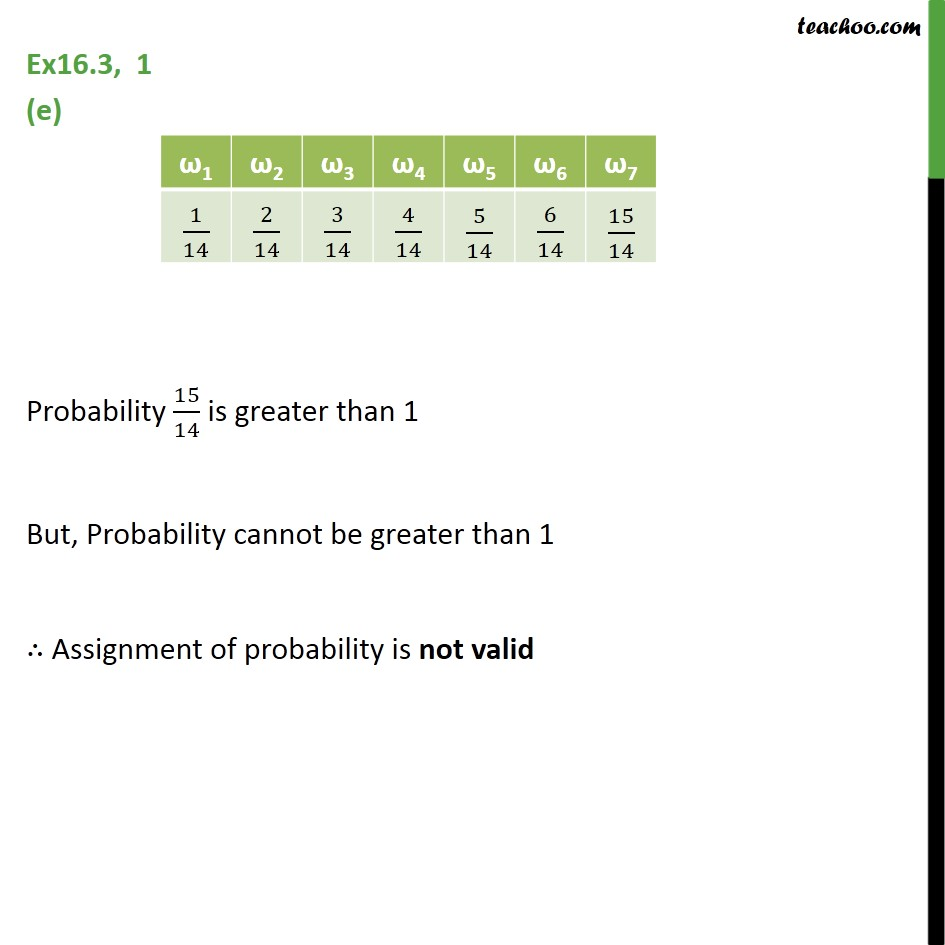Learn in your speed, with individual attention - Teachoo Maths 1-on-1 Class

### Transcript

Ex16.3, 1 Which of the following can not be valid assignment of probabilities for outcomes of sample space S = { ω1, ω2, ω3, ω4, ω5, ω6, ω7,} (a) S = { ω1, ω2, ω3, ω4, ω5, ω6, ω7,} Sum of probability = ω1 + ω2 + ω3 + ω4 + ω5 + ω6 + ω7 = 0.1 + 0.01 + 0.05 + 0.03 + 0.01 + 0.2 + 0.6 = 1.00 ∴ Assignment of probability is valid Ex16.3, 1 (b) Sum of probability = ω1 + ω2 + ω3 + ω4 + ω5 + ω6 + ω7 = 1﷮7﷯ + 1﷮7﷯ + 1﷮7﷯ + 1﷮7﷯ + 1﷮7﷯ + 1﷮7﷯ + 1﷮7﷯ = 1 ∴ Assignment of probability is valid Ex16.3, 1 (c) Sum of probability = ω1 + ω2 + ω3 + ω4 + ω5 + ω6 + ω7 = 0.1 + 0.2 + 0.3 + 0.4 + 0.5 + 0.6 + 0.7 = 2.8 Sum of probability is greater than 1 ∴ This assignment of probability is not valid Ex16.3, 1 (d) Probability of any event cannot be negative ∴ Assignment of probability is not valid Ex16.3, 1 (e) Probability 15﷮14﷯ is greater than 1 But, Probability cannot be greater than 1 ∴ Assignment of probability is not valid